Mathematics
Mapping Diagram
0

 1 Introduction 2 Mapping Diagram 3 Mapping Function 4 Mapping diagram worksheet 5 Summary 6 FAQs

06 October 2020

## Introduction

Mapping, any formulated way of assigning to each object in one set a particular object in another (or the same) set. The figure developed while mapping is mapping diagram and can help solve complex problems.

Mapping Diagram shows how the elements are paired. It is like a flow chart for a function, showing the input and output values. The first column represents the domain of a function f and the other column for its range. Lines or arrows are designed from domain to range, to represent the relation between any two elements.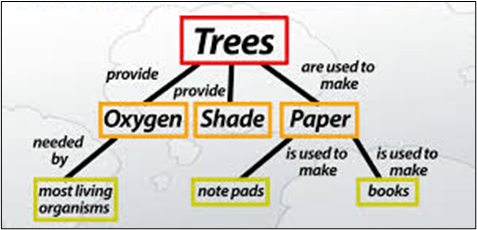## Mapping Diagram

A function is a special type of relation in which each element of the domain is paired with another one element in the range. This pairing can be shown on a Mapping Diagram.

It is similar to a flow chart for a function, showing the input and output values.

### Make a mapping diagram for the relation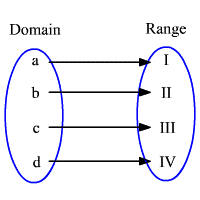A mapping diagram consists of two parallel columns. The first column represents the domain of a function f and the other column for its range. Lines or arrows are designed from domain to range, which represents the relation between any two elements in the mapping diagram.

A function represented by the mapping above in which each element of the range is paired with exactly one element of the domain is called one-to-one mapping. And such a diagram is one to one mapping diagram. And it is also a mapping diagram function.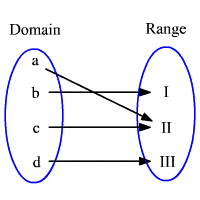In the mapping diagram, the second element of the range identifies more than one element in the domain. If the element(s) in the range that have mapped more than one element in the domain is called many-to-one mapping. And the diagram representing is many to one mapping diagram.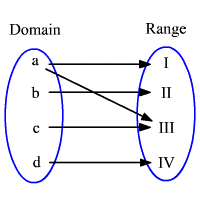In this mapping, the first element in the domain has mapped with more than one element in the range. If one element in the domain is mapped with more than one element in the range, the mapping is called one-to-many relation. One-to-many relations are not functions. Such a diagram is called one to many mapping diagram.

## Mapping Function

Let us see mapping diagram function and function mapping in a little more depth now.

If A and B are two non-empty sets, then a relation‘ from set A to set B is said to be a function or mapping, or mapping function.

• If every element of set A is associated with a unique element of set B.
• Function “ f “ from A to B is denoted by f: A → B.
• If “ f “ is a function from A to B and x ∈ A, and then f ( x ) ∈ B and f ( x ) is called the image of x under f, and x is called the preimage of f ( x ) under “F”.

Note:

• Every element of A must have an image in B. Adjoining figure does not constitute a mapping since the element d in set A is not associated with any element of set B.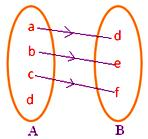• No one element of A must have more than one image in mapping function.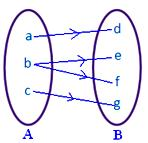Different elements of A have the same image in B.

The adjoining figure represents a mapping function.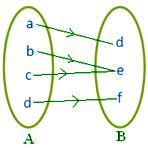### Point to Remember

Every mapping is a relation but every relation is not a mapping.

### Function as a special kind of relation:-

Quickly recall and review the function as a special kind of relation suppose, A and B are two non-empty sets, then a rule ' f ' that associates each element of A with a different element of B is called a function or a mapping from A to B.

Whether ' f ' is a mapping from A to B,

we express it as f: A → B

We can read it as ' f ' is a function from A to B.

If “ f “ is a function from A to B and x ∈ A and y ∈ B, then we can say that y is the image of element x under the function ' f ' and denote it by f (x).

Accordingly, we write it as y = f (x)

Here, element x is called the preimage of y.

Thus, for a function from A to B.

• A and B should be non-empty.
• Each element of A should have an image in B.
• No one element of ' A ' should have more than one image in ' B ’.

Note:

• Two or more images of A may have the same image in B.
• If f: x → y it means that under the function of ' f ' from A to B, an element x of A has image y in B.
• It is mandatory that every f image is in B but there may be some elements in B which are not f images of any element of A.

## Summary

A mapping diagram consists of two parallel columns. The first column represents the domain of a function f and the other column for its range. Lines or arrows.

Mapping Diagram to imagine Complex Analysis illustrates the use of mapping diagrams in the analysis of functions of a complex variable.

In the article, we saw mapping diagram, mapping function, mapping sets, mapping diagram worksheet, and making mapping diagram for the relation.

Written by Neha Tyagi

## What is Set Mapping?

Mapping, any prescribed way of assigning to each object in one set a particular object in another (or the same) set. Mapping is a collection of objects, such as all whole numbers, all the points on a line, or all those inside a circle.

## Is every function a mapping?

All functions are maps but not all maps are functions. A function is a map from one set to another set. However, there are collections that do not obey the set axioms and are therefore not sets.

## What is the difference between relation and mapping?

If A and B are two non-empty sets, then a relation from set A to set B is said to be a function or mapping If every element of set A is associated with a unique element of set B.

## Is a circle a function?

No, a circle is a two-dimensional shape. No. This formula used to describe a circle is an equation, not one function. it has a set of inputs a function must have at most one output.